Search Results
• #### A transfer function is given by H(s) = 40s (s + 10) (s +20) (s +40)...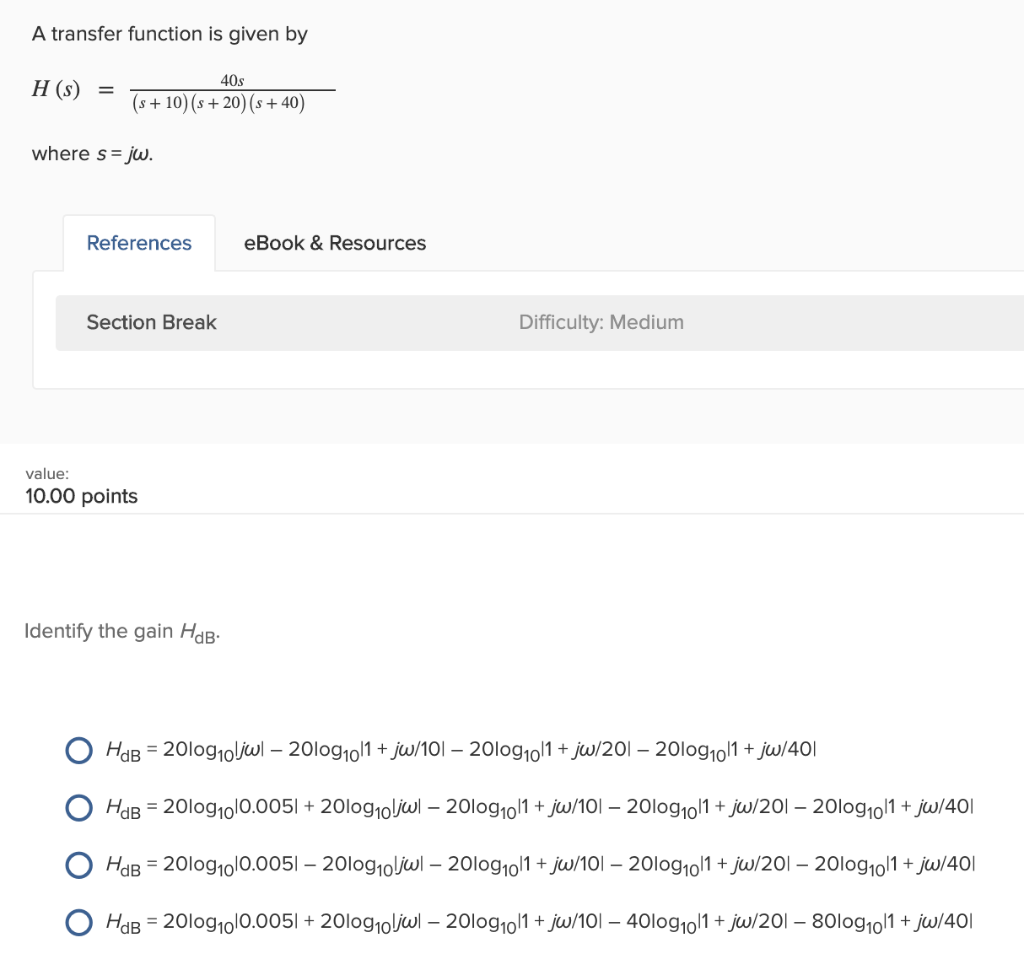A transfer function is given by H(s) = 40s (s + 10) (s +20) (s +40) where s= jw. References eBook & Resources Section Break Difficulty: Medium value: 10.00 points Identify the gain HdB. HdB = 20log oljwl – 2010g10/1 + jw/101 – 2010g10/1 + jw/201 – 2010g1011 + jw/40|| HdB = 2010g1|0.0051 + 20log10jwl – 2010g1011 + jw/101 –...

• #### 15. Consider the following reaction. 2Al (s) + Fe2O3 (s) → Al2O3 (s) + 2Fe (s)...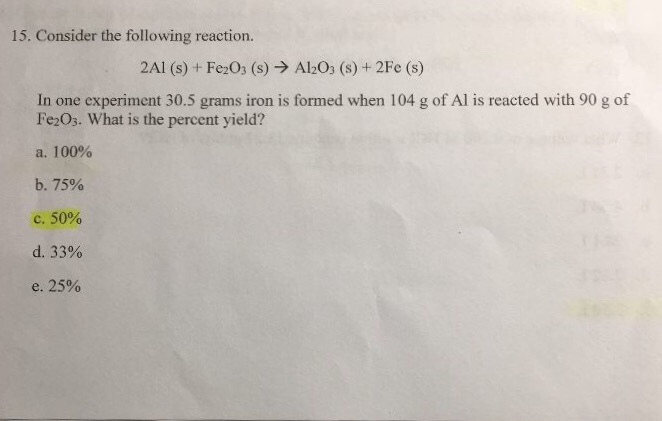15. Consider the following reaction. 2Al (s) + Fe2O3 (s) → Al2O3 (s) + 2Fe (s) In one experiment 30.5 grams iron is formed when 104 g of Al is reacted with 90 g of Fe2Os. What is the percent yield? a. 100% b. 75% C. 50% d. 33% e. 25%

• #### Let I represent an execution of init(s), W of wait(s), and S of signal(s). Then, for...

Let I represent an execution of init(s), W of wait(s), and S of signal(s). Then, for example, IWWS represents the sequence of calls init(s), wait(s), wait(s), and signal(s) by some processes in an operating system. For each of the following sequences of calls, state the value of s and the number of processes blocked after the last call in the...

• #### H(S)= S + S + 25 s'+ 100S Perform a stability analysis of the system. Draw...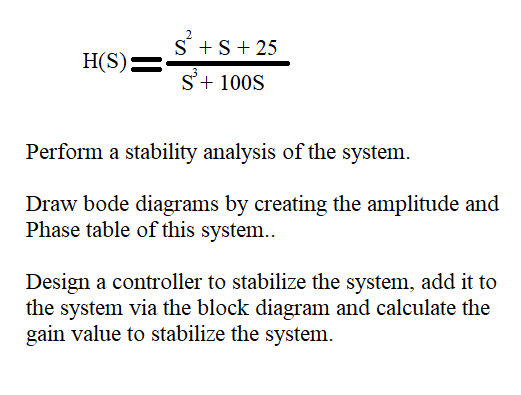H(S)= S + S + 25 s'+ 100S Perform a stability analysis of the system. Draw bode diagrams by creating the amplitude and Phase table of this system.. Design a controller to stabilize the system, add it to the system via the block diagram and calculate the gain value to stabilize the system.

• #### Given the systems below, sketch the root locus: R(S) K C(s) s(s+2)(s+5)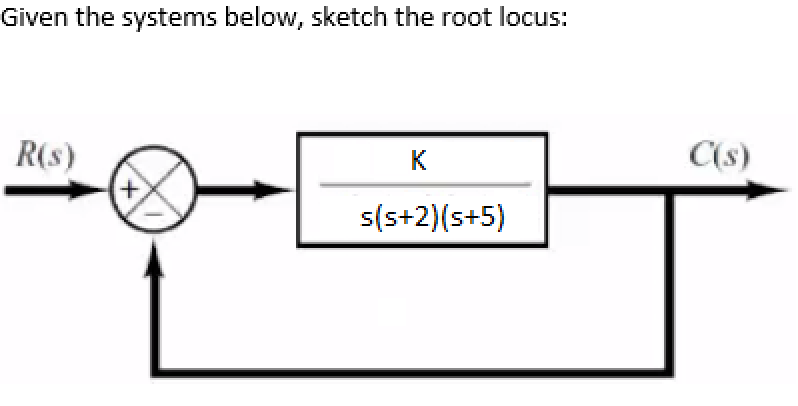Given the systems below, sketch the root locus: R(S) K C(s) s(s+2)(s+5)

• #### 10s(s+20) 3 H(s) = 7 (s +1)(s? +60s + 400) S = 10 (i) Sketch the...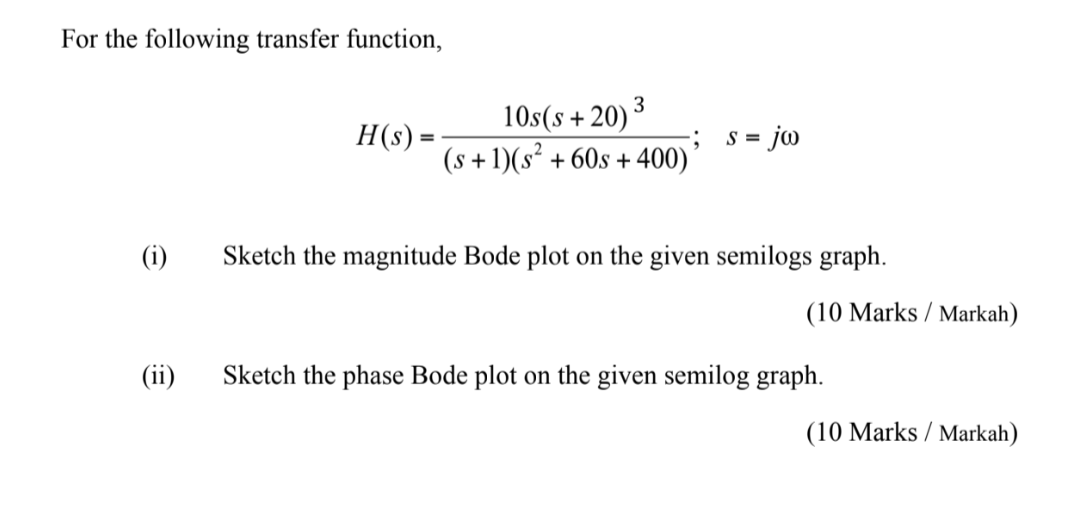10s(s+20) 3 H(s) = 7 (s +1)(s? +60s + 400) S = 10 (i) Sketch the magnitude Bode plot on the given semilogs graph. (10 Marks / Markah) (ii) Sketch the phase Bode plot on the given semilog graph. (10 Marks / Markah)

• #### Problem 4: Given the following function, answer the following questions s +4 H(s) = s(s+10) s...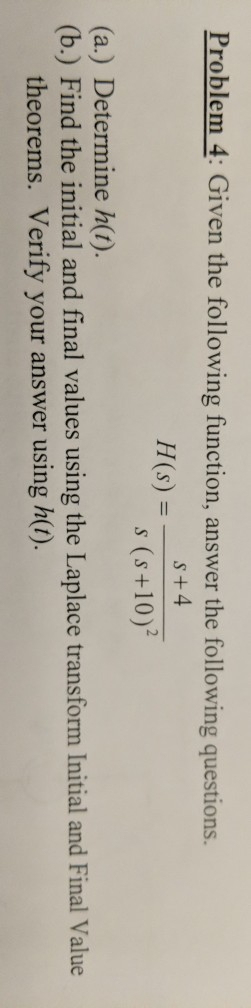Problem 4: Given the following function, answer the following questions s +4 H(s) = s(s+10) s (s+10)2 (a.) Determine h(). (b.) Find the initial and final values using the Laplace transform Initial and Final Value theorems. Verify your answer using h().

• #### (IV) Consider the following block diagram W (s) Y (s) E(s) C1 (s) R (s) P...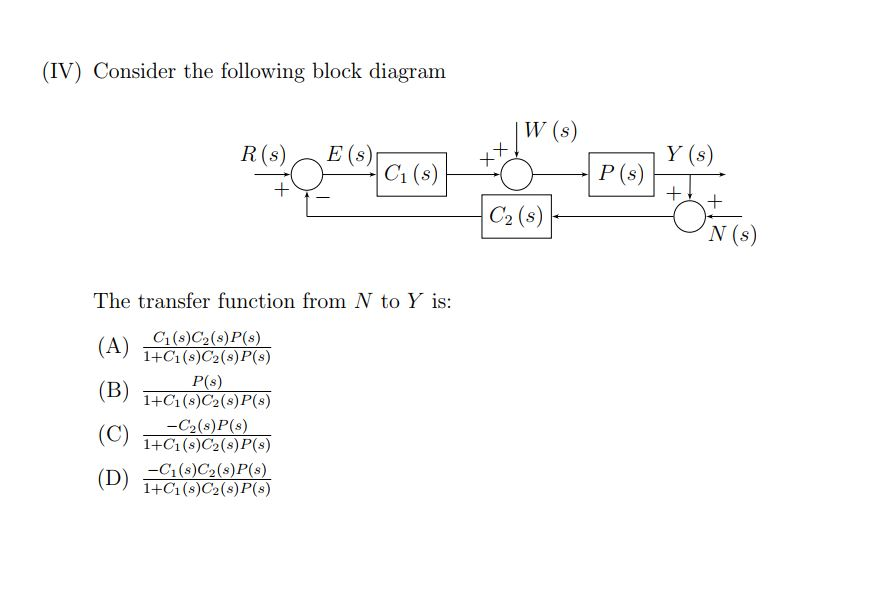(IV) Consider the following block diagram W (s) Y (s) E(s) C1 (s) R (s) P (s) + C2 (s) N (s) The transfer function from N to Y is: C1(s)C2(s)P(s) 1+C1(s)C2(s)P(s) (A) P(s) 1+C1 (s)C2(s)P(s) -C2(s) P(s) (C) 1+C1(s)C2(s)P(s) (B) -C1(s)C2(s)P(s) 1+C1(s)C2(s)P(s) (D)

• #### balance this equ Mg(s) + N2(g) + O2(g) → MgO(s) + Mg3N2(s) MgO(s) + Mg3N2(s) +...

balance this equ Mg(s) + N2(g) + O2(g) → MgO(s) + Mg3N2(s) MgO(s) + Mg3N2(s) + H2O(l) → MgO(s) + Mg(OH)2 (s) + NH3(g) MgO(s) + Mg(OH)2 (s) → MgxOy(s) + H2O(g)

• #### find Consider the Transfer Function Shown Below: G(S) = (s +2) s(s + 3)(s + 5)2...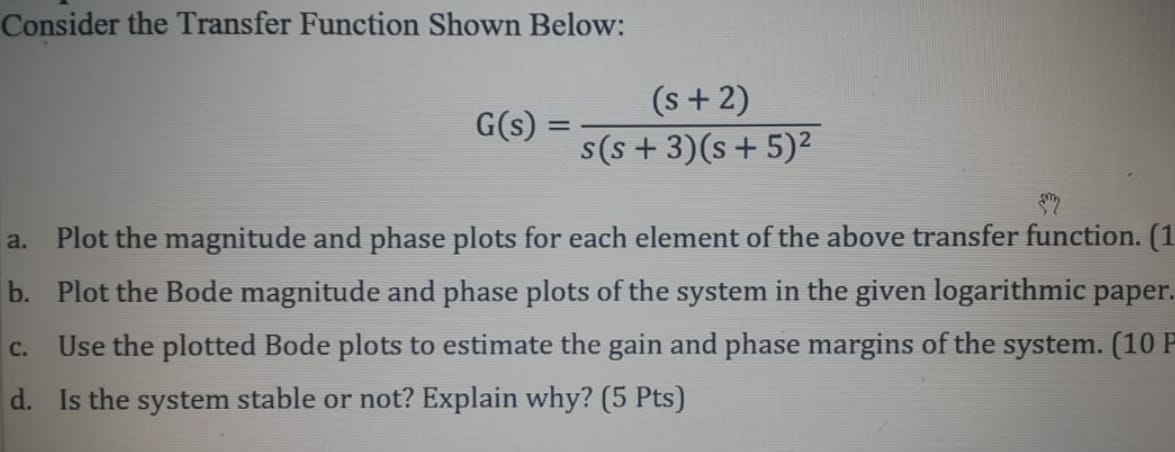find Consider the Transfer Function Shown Below: G(S) = (s +2) s(s + 3)(s + 5)2 a. Plot the magnitude and phase plots for each element of the above transfer function. (1 b. Plot the Bode magnitude and phase plots of the system in the given logarithmic paper. Use the plotted Bode plots to estimate the gain and phase margins...